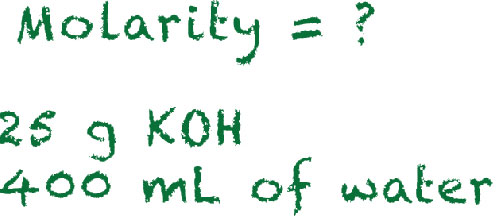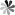Molarity Calculator
Need Assistance?
+
• UK: +

# Molarity Calculator

### Calculate Mass Required for Molar Solution

Our molarity calculator is a useful tool that helps you calculate the:

• mass of a compound required to prepare a solution of known volume and concentration
• volume of solution required to dissolve a compound of known mass to a desired concentration
• concentration of a solution resulting from a known mass of compound in a specific volume

### Calculate now!

g/mol
g* Our calculator is based on the following equation as mass, molar concentration, volume, and formula weight are related to each other:
Mass (g) = Concentration (mol/L) x Volume (L) x Molecular Weight (g/mol)

### Definition

• * Mass (m) is the amount of matter present in a substance. The value is constant and, unlike weight, is not affected by gravity.
• * Formula weight (F.W.) is the sum of the atomic weights of all atoms in a given empirical formula.
• * Molar concentration (also known as Molar concentration) is the amount of a solute present in one unit of a solution. It can be denoted by the unit M, molar.
Online InquiryLoading ......